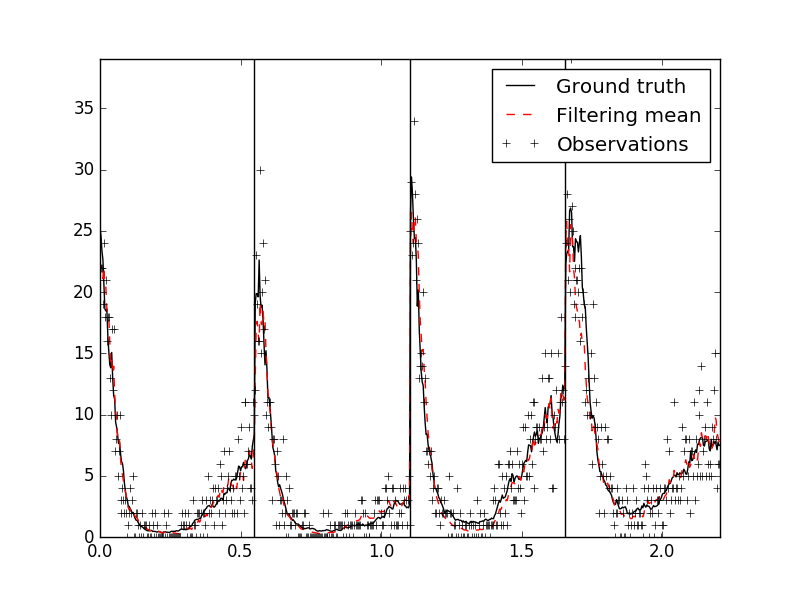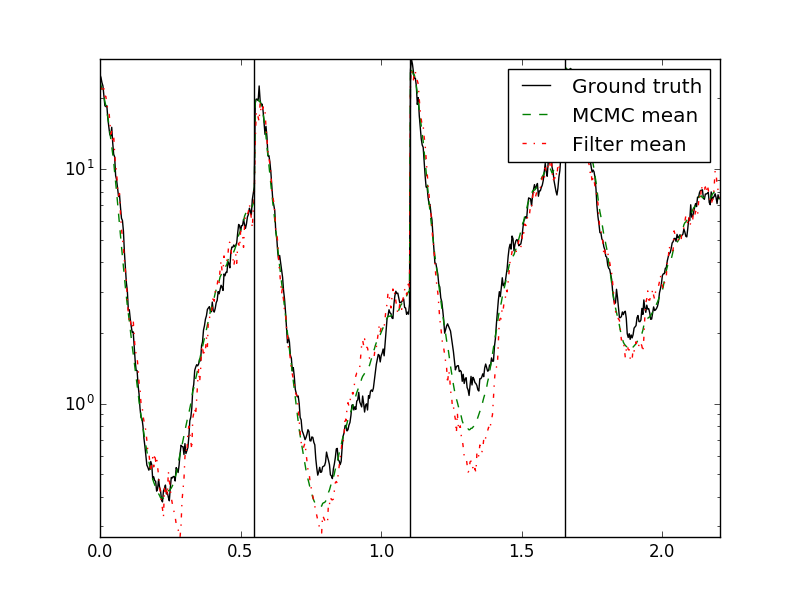# Rao-Blackwellized particle filters for quasiperiodic Gaussian processes

Summary: Notes about implementation of a Rao-Blackwellized particle filter for approximate inference with quasiperiodic Gaussian processes and a Poisson observation model. This kind of model could be applied, for example, to predict the number customers arriving at specific time intervals, when the arrival intensity is time-varying and has daily, weekly etc. seasonality. Python implementation and an experiment with artificial data are available in an accompanying GitHub repository.

## 1   Acknowledgments

The work documented in this post is a sidetrack of my PoDoCo project, which is funded by the Finnish Cultural Foundation and during which I have been a visitor at Aalto University, School of Electrical Engineering, Department of Electrical Engineering and Automation. Thanks!

## 2   Introduction

Periodic Gaussian processes may be used to model latent processes with seasonality, say, a pattern that repeats every day or every week. Quasiperiodic Gaussian processes extend the model so that the process does not repeat forever but changes slowly over time. Solin and Särkkä [SOL2014] describe how to (approximately) represent periodic and quasiperiodic Gaussian processes in the linear-Gaussian state-space model framework. When the observation model is assumed to be such that the observations are linear functions of the latent process with Gaussian noise, this formulation then enables using the Kalman filter and related algorithms to perform Bayesian inference about the latent process.

In this post, I am interested in the case where the observation model is not linear-Gaussian (specifically, Poisson observation models). In these cases, the conditional distribution of the latent process given the observations is not Gaussian and may not have a closed-form at all. In state-space modeling, particle filters (sequential Monte Carlo) are used to perform inference in the general cases where analytic solutions do not exist. In the present case, the state of the state-space-form-of-quasiperiodic-GP is multidimensional (intuition: the latent process is not Markovian, so more information than the current value of the process needs to be carried) while the observations depend on the values of the actual latent process. This enables the use of a Rao--Blackwellized particle filter (RBPF, also known as marginalized particle filter): the filter needs to sample only the values of the latent process and Kalman filtering can be use to keep track of the conditional distribution of the state conditional on the sampled process.

I have not performed a thorough literature survey - based on a quick glance, Reece et al. [REE2014] use a RBPF in connection with their periodic framework. However, they use it to sample a discrete component of the dynamic process, while here the dynamic process is linear-Gaussian and the particle filtering is used due to a nonlinear observation model.

## 3   The algorithm

The conversion of Gaussian processes to state-space models is implemented in GPy which I use here as a black box. So, the algorithm starts from the following model specification:

\begin{align*} \mathbf{x}_0 & \sim \mathrm{N}(\mathbf{m}_0,~\mathbf{P}_0) \\ \mathbf{x}_{k} \mid \mathbf{x}_{k-1} & \sim \mathbf{N}(\mathbf{A}_k\,\mathbf{x}_{k-1},~\mathbf{Q}_k), \\ y_k \mid \mathbf{x}_k & \sim p(y_k \mid h_k), \\ h_k &= \mathbf{H}\,\mathbf{x}_k, \end{align*}

with the joint distribution factorizing as the product of the aforementioned conditional distributions. In this work, I assumed

\begin{equation*} y_k \mid h_k \sim \mathrm{Poisson}(\mathrm{exp}(h_k + \mu)), \end{equation*}

where the offset $\mu$ is a known constant.

The full particle filter algorithm is then

1. Initialize the filter: $\mathbf{m}^{(i)} := \mathbf{m}^{(i)}_0$, $W^{(i)}:=1/N,~ i=1,\ldots,N$ and $\mathbf{P}:=\mathbf{P}_0$.
2. For $k=1,2,\ldots$
1. Prediction step: $\mathbf{m}^{(i)}:=\mathbf{A}_k\,\mathbf{m}^{(i)},~i=1,\ldots,N$ and $\mathbf{P} = \mathbf{A_k}\,\mathbf{P}\,\mathbf{A_k}^{T} + \mathbf{Q}_k$
2. Compute the conditional variance of $h_k$: $S := \mathbf{H}\,\mathbf{P}\,\mathbf{H}^{T}$
3. Sampling step: sample $h^{(i)}_k \sim \mathrm{N}(\mathbf{H}\,\mathbf{m},~\mathbf{S}),~i=1,\ldots,N$
4. Weight update step: $W^{(i)}:= W^{(i)}\,p(y_k \mid h^{(i)}_k)$, normalize weights
5. Kalman filter update step: update $\mathbf{m}^{(i)},i=1,\ldots,N$ and $\mathbf{P}$ by conditioning on $h^{(i)}_k$
6. Possible resampling step

Here, I resampled at every step using stratified resampling.

I also implemented a Particle Independent Metropolis-Hastings MCMC sampler, see [AND2010], to sample from the joint smoothing distribution $p(h_{1:T} \mid y_{1:T})$ utilizing this Rao-Blackwellized particle filter.

## 4   Demonstration

This demonstration is based on simulating artificial data consisting of 4 days, with each observed Poisson count corresponding to a 5 minute interval. During each day, 159 consecutive intervals are observed, so that there is an unobserved period consisting of the remaining 129 intervals in each day.

The latent process is specified to be a Gaussian process with covariance

\begin{equation*} \kappa(t,t') = \kappa_1(t,t')\,\kappa_2(t,t') + \kappa_3(t,t'), \end{equation*}

with

• $\kappa_1$: Standard periodic covariance, lengthscale 0.5, variance 2,
• $\kappa_2$: Matérn 3/2 covariance, lengthscale 10, variance 1,
• $\kappa_3$: Exponential covariance, lengthscale 0.3, variance 0.15,

where the lengthscales are on the scale of days. The offset is $\mu=0.5$. The data was simulated from this process.

The particle filter was assumed to know all parameters exactly. 200 particles was used. See the figure below for a plot of the ground-truth intensity ($\mathrm{exp}(h(t)+\mu)$), the filtering mean, and the ground truth. The vertical lines refer to the breaks between days (the tickmarks of the x-axis count days from the beginning, excluding the breaks, sorry).And the figure below for the MCMC results (200 particles, 1000 samples, first 250 discarded as warmup).Finally, the Table below shows the RMSE of the estimates of the Poisson mean based on the model a priori, the raw observations, the filter, and the MCMC smoother. As expected, the smoother is better than the filter which is better than either using the observations only or the prior only.

 Prior Observations Filter MCMC RMSE: 5.9 2.3 1.2 0.8